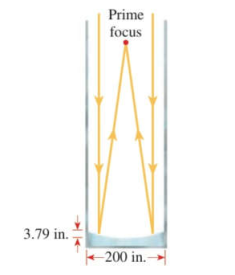Chapter 12.1, Problem 64E### Algebra and Trigonometry (MindTap ...

4th Edition
James Stewart + 2 others
ISBN: 9781305071742

#### Solutions

Chapter
Section### Algebra and Trigonometry (MindTap ...

4th Edition
James Stewart + 2 others
ISBN: 9781305071742
Textbook Problem

# APPLICATIONS 64. Reflecting Telescope The Hale telescope at the Mount Palomar Observatory has a 200 − in mirror, as shown in the figure. The mirror is constructed in a parabolic shape that collect slight from the stars and focuses it at the prime focus. that is the focus of the parabola. The mirror is 3.79 in. deep at its center. Find the focal length of this parabolic mirror, that is, the distance from the vertex to the focus.To determine

To find:

Thefocal length of parabolic mirror inside the Hale telescope.

Explanation

Given:

The Hale telescope at the Mount Palomar observatory is as shown in figure (1).

Figure (1)

The shape of mirror is a parabola and has diameter of 200in. and a depth of 3.79in. It collects the light from stars and focuses it to the prime focus.

Concept:

The general equation of a parabola with vertical axis where vertex V(0,0) and focus at F(0,p) is given by,

x2=4py (1)

Where y=p is the directrix.

1) If p<0, then parabola opens downward.

2)If p>0, then parabola opens upward.

Focal length is the distance between vertex of parabola and the point where reflected rays intersect, i.e. focus. In equation x2=4py, p is the focal length

### Still sussing out bartleby?

Check out a sample textbook solution.

See a sample solution

#### The Solution to Your Study Problems

Bartleby provides explanations to thousands of textbook problems written by our experts, many with advanced degrees!

Get Started